Start typing, then use the up and down arrows to select an option from the list.# Clausius-Clapeyron Equation Concept 3

Jules Bruno
320views
In this section of the classiest clapper on equation, we're going to take a look at its 2.4. Now we use this form of the equation when two temperatures and or two pressures are mentioned. And we're going to say that we need to remember when given normal boiling point Pressure equals 760 tour or 760 of mercury. This is known as our normal pressure. Now with the two point of the classiest copper an equation, we now have L N A P two over P one equals negative delta H V over R times one over T two minus one over T one. Here we're gonna say ours our gas constant, it's 8.314 jewels over moles times K P equals our favorite pressure again in tours or millimeters of mercury. Delta H Vape is our entropy of vaporization, which is in jewels per mole. And the temperatures here have to be in units of kelvin. So keep this in mind when we're talking about the classiest clapper on equation and were given to temperatures and or two pressures, we have to use a two point form of the equation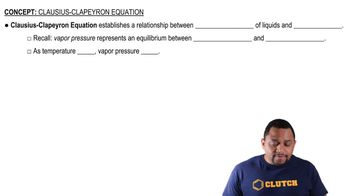00:59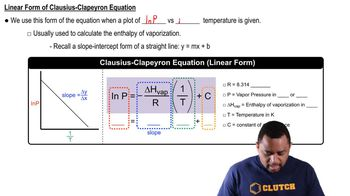02:57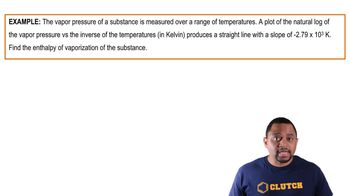01:37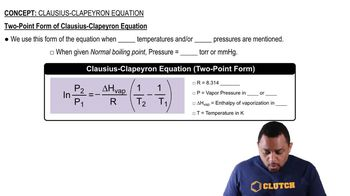01:13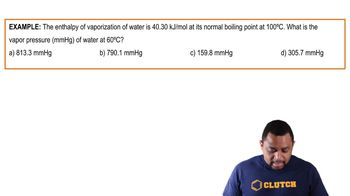03:37